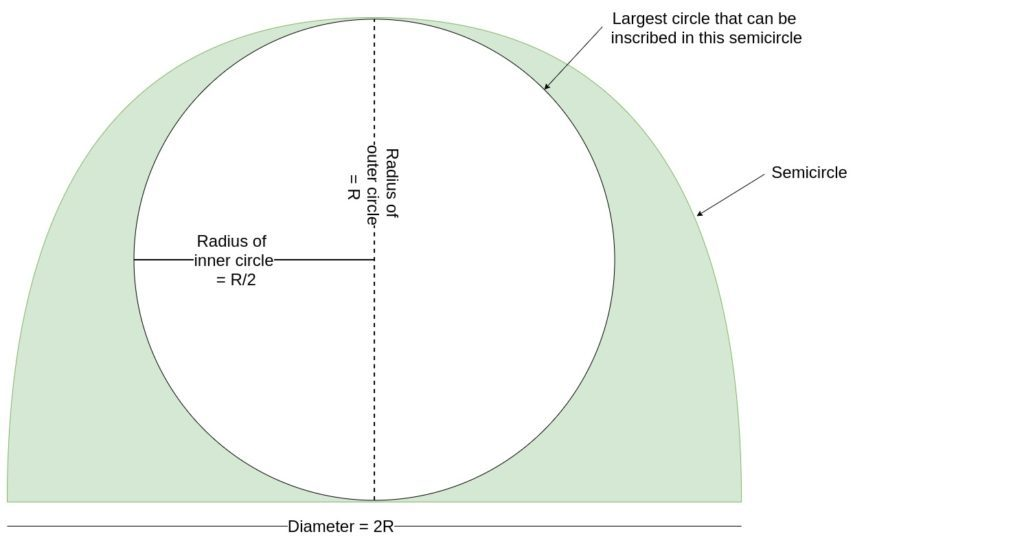# Area of largest Circle that can be inscribed in a SemiCircle

Given a semicircle with radius R, the task is to find the area of the largest circle that can be inscribed in the semicircle.

Examples:

```Input: R = 2
Output: 3.14

Input: R = 8
Output: 50.24
```

## Recommended: Please try your approach on {IDE} first, before moving on to the solution.

Approach: Let R be the radius of the semicircle

1. For Largest circle that can be inscribed in this semicircle, the diameter of the circle must be equal to the radius of the semi-circle.
2. So, if the radius of the semi-circle is R, then the diameter of the largest inscribed circle will be R.
3. Hence the radius of the inscribed circle must be R/2
4. Therefore the area of the largest circle will be
```Area of circle = pi*Radius2
= pi*(R/2)2

since the radius of largest circle is R/2
where R is the radius of the semicircle
```Below is the implementation of the above approach:

## C++

 `// C++ Program to find the biggest circle ` `// which can be inscribed within the semicircle ` ` `  `#include ` `using` `namespace` `std; ` ` `  `// Function to find the area ` `// of the circle ` `float` `circlearea(``float` `R) ` `{ ` ` `  `    ``// Radius cannot be negative ` `    ``if` `(R < 0) ` `        ``return` `-1; ` ` `  `    ``// Area of the largest circle ` `    ``float` `a = 3.14 * R * R / 4; ` ` `  `    ``return` `a; ` `} ` ` `  `// Driver code ` `int` `main() ` `{ ` `    ``float` `R = 2; ` `    ``cout << circlearea(R) << endl; ` ` `  `    ``return` `0; ` `} `

## Java

 `// Java Program to find the biggest circle ` `// which can be inscribed within the semicircle ` `class` `GFG  ` `{ ` `     `  `    ``// Function to find the area ` `    ``// of the circle ` `    ``static` `float` `circlearea(``float` `R) ` `    ``{ ` `     `  `        ``// Radius cannot be negative ` `        ``if` `(R < ``0``) ` `            ``return` `-``1``; ` `     `  `        ``// Area of the largest circle ` `        ``float` `a = (``float``)((``3.14` `* R * R) / ``4``); ` `     `  `        ``return` `a; ` `    ``} ` `     `  `    ``// Driver code ` `    ``public` `static` `void` `main (String[] args)  ` `    ``{ ` `        ``float` `R = ``2``; ` `        ``System.out.println(circlearea(R)); ` `    ``} ` `} ` ` `  `// This code is contributed by AnkitRai01 `

## Python3

 `# Python3 Program to find the biggest circle ` `# which can be inscribed within the semicircle ` ` `  `# Function to find the area ` `# of the circle ` `def` `circlearea(R) : ` ` `  `    ``# Radius cannot be negative ` `    ``if` `(R < ``0``) : ` `        ``return` `-``1``; ` ` `  `    ``# Area of the largest circle ` `    ``a ``=` `(``3.14` `*` `R ``*` `R) ``/` `4``; ` ` `  `    ``return` `a; ` ` `  `# Driver code ` `if` `__name__ ``=``=` `"__main__"` `: ` ` `  `    ``R ``=` `2``; ` `    ``print``(circlearea(R)) ; ` `     `  `# This code is contributed by AnkitRai01 `

## C#

 `// C# Program to find the biggest circle ` `// which can be inscribed within the semicircle ` `using` `System; ` ` `  `class` `GFG  ` `{ ` `     `  `    ``// Function to find the area ` `    ``// of the circle ` `    ``static` `float` `circlearea(``float` `R) ` `    ``{ ` `     `  `        ``// Radius cannot be negative ` `        ``if` `(R < 0) ` `            ``return` `-1; ` `     `  `        ``// Area of the largest circle ` `        ``float` `a = (``float``)((3.14 * R * R) / 4); ` `     `  `        ``return` `a; ` `    ``} ` `     `  `    ``// Driver code ` `    ``public` `static` `void` `Main (``string``[] args)  ` `    ``{ ` `        ``float` `R = 2; ` `        ``Console.WriteLine(circlearea(R)); ` `    ``} ` `} ` ` `  `// This code is contributed by AnkitRai01 `

Output:

```3.14
```

Don’t stop now and take your learning to the next level. Learn all the important concepts of Data Structures and Algorithms with the help of the most trusted course: DSA Self Paced. Become industry ready at a student-friendly price.

My Personal Notes arrow_drop_upCheck out this Author's contributed articles.

If you like GeeksforGeeks and would like to contribute, you can also write an article using contribute.geeksforgeeks.org or mail your article to contribute@geeksforgeeks.org. See your article appearing on the GeeksforGeeks main page and help other Geeks.

Please Improve this article if you find anything incorrect by clicking on the "Improve Article" button below.

Improved By : AnkitRai01